Next: The Rayleigh quotient trick Up: The Trust-Region subproblem Previous: Initial values of and   Contents

# How to find a good approximation of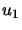: LINPACK METHODis the unit eigenvector corresponding to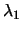. We need this vector in the hard case (see the paragraph containing equation 4.15 ). Sinceis the eigenvector corresponding to, we can write: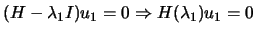We will try to find a vectorwhich minimizes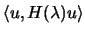. This is equivalent to find a vector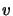which maximize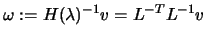. We will choose the component ofbetween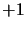and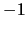in order to make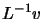large. This is achieved by ensuring that at each stage of the forward substitution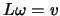, the sign ofis chosen to make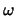as large as possible. In particular, suppose we have determined the first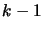components ofduring the forward substitution, then the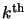component satisfies: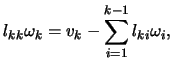and we pick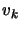to be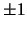depending on which of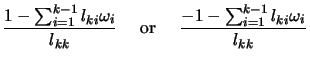is larger. Having found,is simply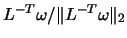. The vectorfound this way has the useful property that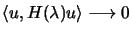as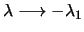Next: The Rayleigh quotient trick Up: The Trust-Region subproblem Previous: Initial values of and   Contents
Frank Vanden Berghen 2004-04-19